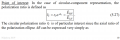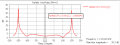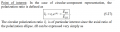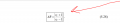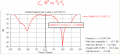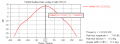# physical logic of axial ratio measurement using Co and cross polarization

#### yef smith

Joined Aug 2, 2020
293
Hello,there is an intresting youtube manual regarding measuring axial ratio in anechoic chamber shown bellow.
in the video i think they have a circularily polarzied antenna which is being tested by linear polarised antenna.
They rotate the linear antenna ,thus changing the polarization of the reference antenna.
So if we calculate the polarization loss factor of linear with respect to circularly polarised.
by the formula bellow it remains the same(PLF).

In CST we have CO-polarization and Cross polarization,so given the manual in the link bellow
our R_C is Amplitude in RH/ Amplitude in LH
In the results shown bellow R_C=R_H/L_H=7.8-(-11)=18dB
So out AXIAL ratio will be 0dB almost.
The same thing will happen if we use linear polarization case.
its CO polarization will be much higher then the cross polarization and by this formula We will get AXIAL ratio of 0dB Even in linear.
Where did i go wrong using this formula?
Thanks.